# Class 12 Maths NCERT Solutions for Chapter 4 Determinants Exercise 4.1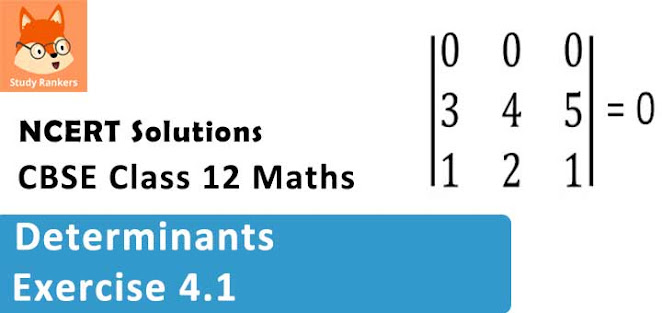### Determinants Exercise 4.1 Solutions

1. Evaluate the determinants in Exercises 1 and 2.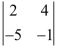Solution= 2(-1) - 4(-5) = -2 +20 = 18

2. Evaluate the determinants in Exercises 1 and 2.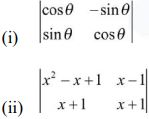Solution

(i)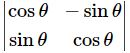=  (cos θ)(cos θ) − (−sin θ)(sin θ) = cos2 θ+ sin2 θ = 1

(ii)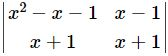= (x2 − x + 1)(x + 1) − (x − 1)(x + 1)
x3 − x2 + x + x2 − x + 1 − (x2 − 1)
x3 + 1 − x2 + 1
x3 − x2 + 2

3. If A =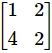then show that |2A| = 4|A|

Solution

The given matrix is A =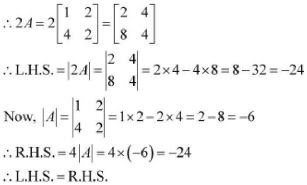4. If A =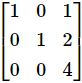then show that |3A| = 27|A| .

Solution

The given matrix is A =It can be observed that in the first column, two entries are zero. Thus, we expand along the first column (C1) for easier calculation.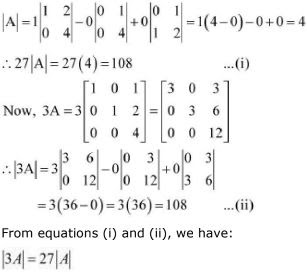Hence, the given result is proved.

5. Evaluate the determinants

(i)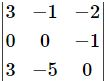(ii)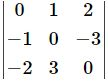(iii)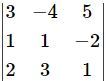(iv)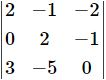Solution

(i) Let A =It can be observed that in the second row, two entries are zero. Thus, we expand along the second row for easier calculation.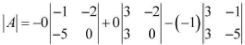= (-15 + 3) = -12
(ii) Let A =By expanding along the first row, we have :
|A| =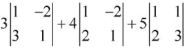= 3(1 + 6) + 4(1 + 4) + 5(3 - 2)
= 3(7) + 4(5) + 5(1)
= 21 + 20 +5
= 46

(iii) Let A =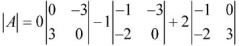= 0 - 1(0 - 6) + 2(-3-0)
= -1(-6) + 2(-3)
= 6 -6 = 0

(iv) Let A =By expanding along the first column, we have :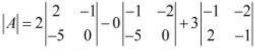= 2(0 - 5) - 0 + 3(1 + 4)
= -10 + 15 = 5

6. If A =, Find |A|

Solution

Let A =By expanding along the first row, we have :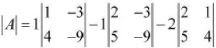= 1(-9 + 12) - 1(-18 + 15) - 2(8 - 5)
= 1(3) - 1(-3) - 2(3)
= 3 + 3 - 6
= 6 - 6  = 0

7. Find values of x, if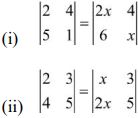Solution

(i)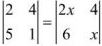⇒ 2× 1 - 5× 4 = 2x × x - 6×4
⇒ 2 - 20 = 2x2 – 24
⇒ 2x2 = 6
⇒ x2 = 3
⇒ x = ±√3

(ii)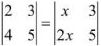⇒ 2× 5 - 3× 4 = x × 5 - 3 × 2x
⇒ 10 - 12 = 5x - 6x
⇒ -2 = -x
⇒ x = 2

8. If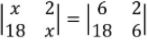, then x is equal to

(A) 6

(B) ± 6

(C) -6

(D) 0

Solution⇒ x2 - 36 = 36 - 36
⇒ x2 - 36 = 0
⇒ x2 = 36
⇒ x = ± 6
Hence, the correct answer is B.